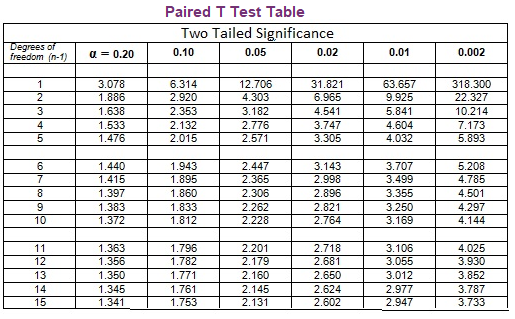# Paired T-Test

In Statistics, a t-test can be represented as a statistical hypothesis test where the test statistic supports a student’s t distribution, if the null hypothesis is established. In Paired T-Test, they compare the means of two groups of observations. The observations must be randomly assigned to each of the two groups so that the difference in response seen is due to the treatment and not because of any other factors. If two samples are given, then the observation of one sample can be paired with the observation of the other sample. This test can be used in making observations on the same sample before and after an event. Now, let us discuss what is paired t-test, its formula, table and the procedure to perform the paired t-test in detail.

## Paired T-Test Definition

The paired t-test gives a hypothesis examination of the difference between population means for a set of random samples whose variations are almost normally distributed. Subjects are often tested in a before-after situation or with subjects as alike as possible. The paired t-test is a test that the differences between the two observations are zero.

Let us assume two paired sets, such as Xi and Yi for i = 1, 2, …, n such that their paired difference are independent which are identically and normally distributed. Then the paired t-test concludes whether they notably vary from each other.

## Paired T-Test Formula

Paired T-test is a test which is based on the differences between the values of a single pair, that is one deducted from the other. In the formula for a paired t-test, this difference is notated as d. The formula of the paired t-test is defined as the sum of the differences of each pair divided by the square root of n times the sum of the differences squared minus the sum of the squared differences, overall n-1.

The formula for the paired t-test is given by

$$t = \frac{\sum d}{\sqrt{\frac{n(\sum d^{2})-(\sum d)^{2}}{n-1}}}$$

Where, Σd is the sum of the differences.

## Paired T-Test Table

Paired T-test table enables the t-value from a t-test to be converted to a statement about significance. The table is given below:## Paired Vs Unpaired T-Test

The similarity between paired and unpaired t-test is that both assume data from the normal distribution.

Characteristics of Unpaired T-Test:

• The two groups taken should be independent.
• The sample size of the two groups need not be equal.
• It compares the mean of the data of the two groups.
• 95% confidence interval for the mean difference is calculated.

Characteristics of Paired T-Test:

• The data is taken from subjects who have been measured twice.
• 95% confidence interval is obtained from the difference between the two sets of joined observations.

### How to Find the paired T-Test

Let us take two sets of data that are related to each other, say X and Y with xi ∈ X, yi ∈ Y. where i = 1, 2,……., n. Follow the steps given below to find the paired t-test.

• Assume the null hypothesis that the actual mean difference is zero.
• Determine the difference di = yi – xi between the set of observation.
• Compute the mean difference.
• Calculate the standard error of the mean difference, which is equal to Sd /√n, where n is the total number, and Sd is the standard deviation of the difference.
• Determine the t-statistic value.
• Refer to the T-distribution table and compare it with the tn-1 distribution. It gives the p-value.

To learn more on Statistics topics, visit BYJU’S – The Learning App and download the app to watch the interactive videos to learn with ease.